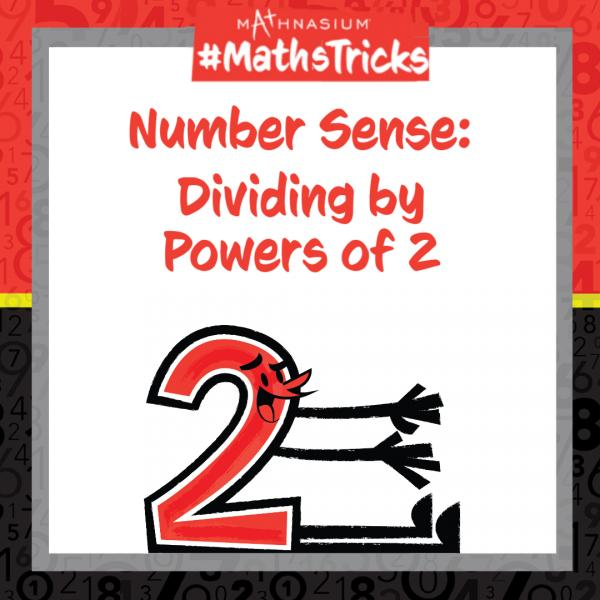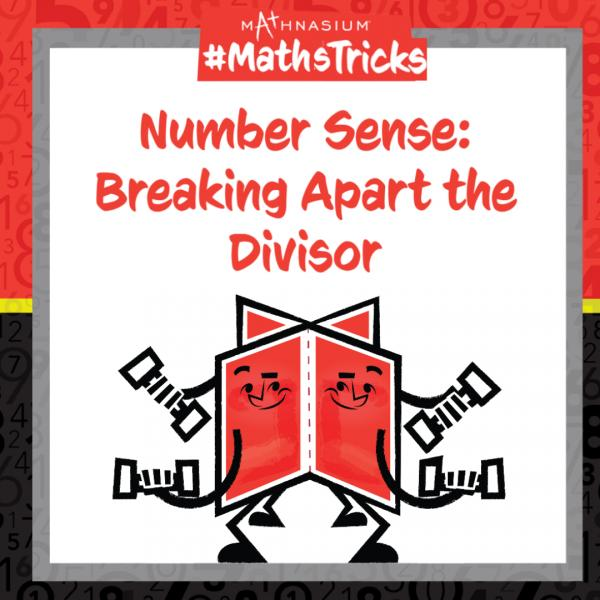# Number Sense Blog### Mathnasium #MathsTricks: Fractions (Comparing Fractions Part 2)

Jun 27, 2022

Welcome to Mathnasium’s Maths Tricks series. Today we are comparing fractions that have the same numerators but have different denominators (the total number of equal parts in the whole). When we compare fractions with the same numerators, we c...### Mathnasium #MathsTricks: Number Sense (Division Part 1)

Jun 27, 2022

Welcome to Mathnasium’s Maths Tricks series. Students are often taught that division is the opposite of multiplication, and therefore should rely on their multiplication facts to divide; this can be difficult when the number to divide by (the divi...### Mathnasium #MathsTricks: Comparing Fractions Part 3

Jun 27, 2022

Welcome to Mathnasium’s Math Tricks series. Today we are comparing fractions to the benchmark number, one half. When comparing fractions, it can be difficult to determine whether they represent values that are greater than or less than one anot...### Mathnasium #MathsTricks: Number Sense (Division Part 2)

Jun 27, 2022

Welcome to Mathnasium’s Maths Tricks series. Today we are using number sense to divide statements where the divisors (the numbers we are dividing by) have more than two factors (numbers which divide evenly into the divisor). Rather than dividing a...### Ask Education: How Deep is the Ocean?

Jun 27, 2022

Our expert team of maths educators and enthusiasts has spent over 40 years developing and refining the most powerful teaching methods and materials into the comprehensive, industry-leading Mathnasium Method™. These “Ask Education” features are a w...

Page 1 of 2, showing 5 records out of 8 total, starting on record 1, ending on 5.

Previous 12